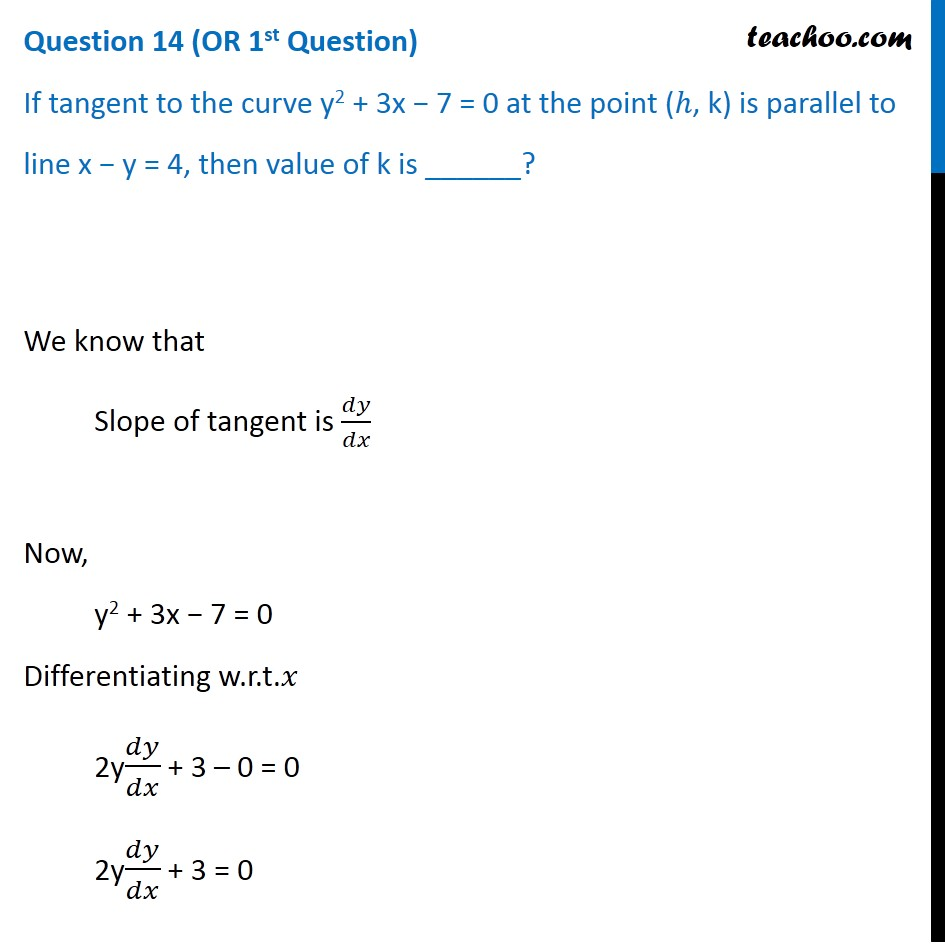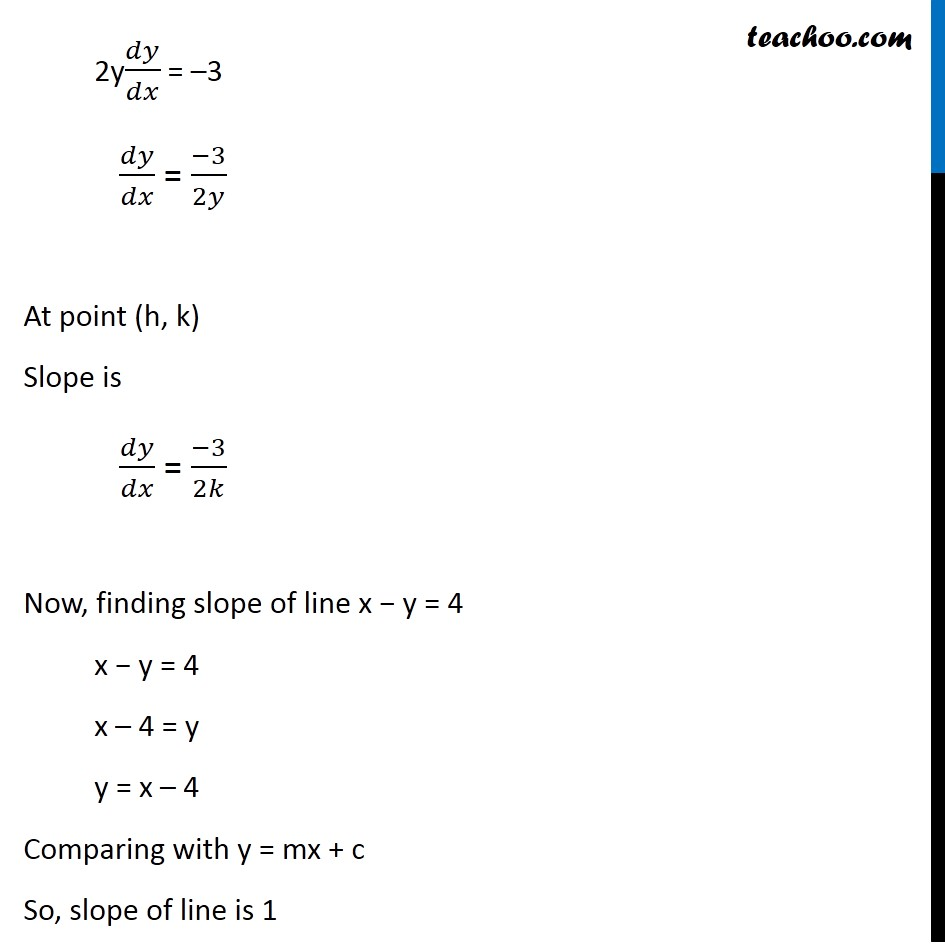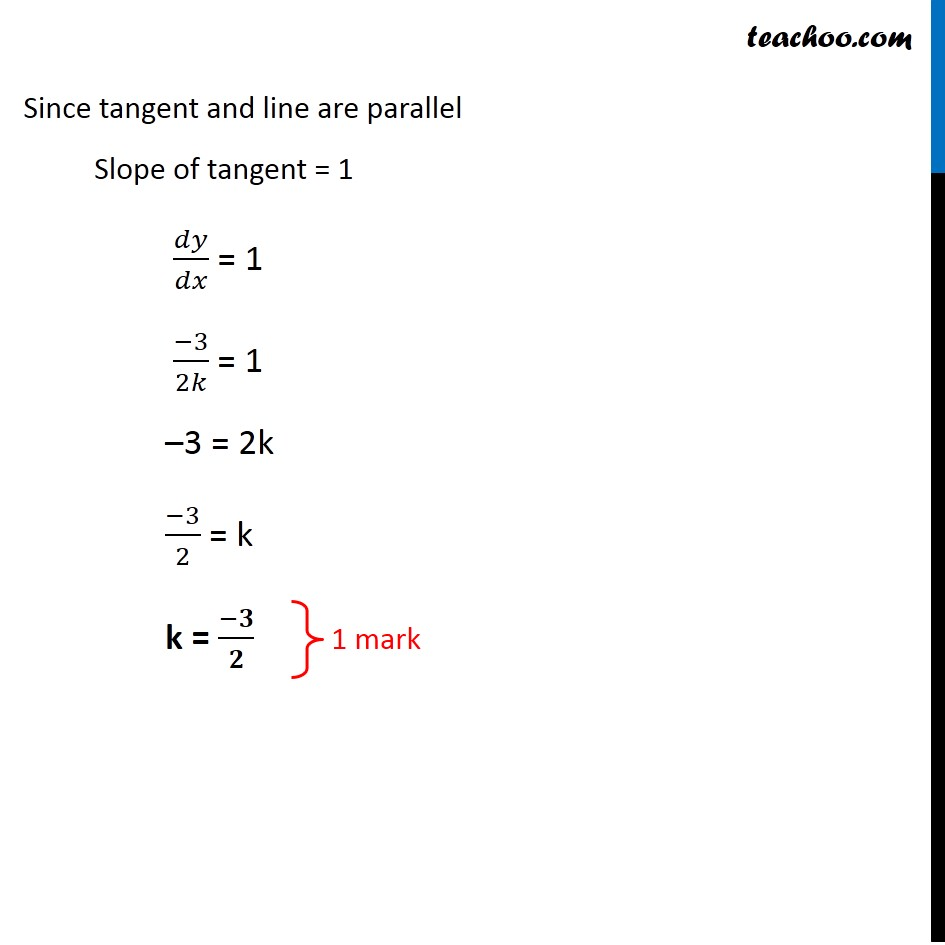CBSE Class 12 Sample Paper for 2020 Boards

Class 12
Solutions of Sample Papers and Past Year Papers - for Class 12 Boards

## If tangent to the curve y 2 + 3x − 7 = 0 at the point (ℎ, k) is parallel to line x − y = 4, then value of k is ______?Learn in your speed, with individual attention - Teachoo Maths 1-on-1 Class

### Transcript

Question 14 (OR 1st Question) If tangent to the curve y2 + 3x − 7 = 0 at the point (ℎ, k) is parallel to line x − y = 4, then value of k is ______? We know that Slope of tangent is 𝑑𝑦/𝑑𝑥 Now, y2 + 3x − 7 = 0 Differentiating w.r.t.𝑥 2y𝑑𝑦/𝑑𝑥 + 3 – 0 = 0 2y𝑑𝑦/𝑑𝑥 + 3 = 0 2y𝑑𝑦/𝑑𝑥 = –3 𝑑𝑦/𝑑𝑥 = (−3)/2𝑦 At point (h, k) Slope is 𝑑𝑦/𝑑𝑥 = (−3)/2𝑘 Now, finding slope of line x − y = 4 x − y = 4 x – 4 = y y = x – 4 Comparing with y = mx + c So, slope of line is 1 Since tangent and line are parallel Slope of tangent = 1 𝑑𝑦/𝑑𝑥 = 1 (−3)/2𝑘 = 1 –3 = 2k (−3)/2 = k k = (−𝟑)/𝟐